# Calculating phase shift

#### Fluke289

Joined Aug 8, 2013
21
I have a simple inductor circuit shown here: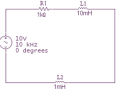I calculated the voltage drops for R1, L1, and L2. For my calculations I calculated:
R1 = 8.23 VP-p @ -34.65 degrees
L1 = 6.17 VP-p @ 55.35 degrees
L2 = 515 mVp-p @ 55.35 degrees

When I add all those up, I get about 10 VP-p with pretty close to 0 degrees phase, so I'm happy.

I decided to build the circuit to see what my actual measurements would be.

For R1 I measured about 8.22 VP-p, but my phase shift is at about -55 degrees. For my L1 and L2, I hardly see any phase shift on the circuit. I understand that there will be differences between calculated values and measured values, but to me this seems like a pretty huge difference.

This is a picture of how I built my circuit while looking at R1. The channel 1 probe is on the left and the channel 2 probe is on the right attached to the resistor.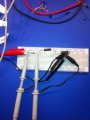This is a screen shot of what I'm reading on my oscilloscope.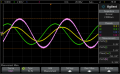I must be doing something wrong, or not understanding something correctly. Any help would be appreciated.

#### WBahn

Joined Mar 31, 2012
26,398
It would be easier to tell what you are doing if your voltage channels were displayed using the same scale.

It appears that you are adding the two voltages, but don't you want to subtract Channel 2 from Channel 1 to get the voltage difference across the resistor?

And isn't the phase angle you are looking for the difference between the Channel 1 signal (i.e., the source serving as your reference) and the voltage difference across the resistor?

#### Fluke289

Joined Aug 8, 2013
21
It would be easier to tell what you are doing if your voltage channels were displayed using the same scale.
I'm sorry, I forgot to set the scale properly before I took the screen shot. Here is an updated screen shot.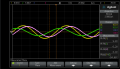It appears that you are adding the two voltages, but don't you want to subtract Channel 2 from Channel 1 to get the voltage difference across the resistor?
To get the voltage drop across the resistor, I'm using Channel 1 as the reference and I'm subtracting Channel 2 from that. The purple Pk-Pk(M) is reading about 8.2V, which is almost exactly what I calculated. I'm assuming that's correct.

And isn't the phase angle you are looking for the difference between the Channel 1 signal (i.e., the source serving as your reference) and the voltage difference across the resistor?
The -55 degree Phase 1 -> 2 that's on the screen shot, as far as I understand is this:

Phase = $$\frac{Delay}{Source 1 Period}$$ X 360

I assume that's the phase angle that I'm looking for.

Here is another screen shot without the phase angle displayed, so that it's easier to read the tick marks on the scope.#### WBahn

Joined Mar 31, 2012
26,398

You calculated the voltage across the resistor to be 8.23 VP-p @ -34.65 degrees.

That is your YELLOW trace, which you used to measure the Vpp of and got 8.16V.

But then you measured the phase angle of the GREEN trace, a trace with a Vpp of 5.6V, and got a phase angle of the -56.04°.

Why do you assume that you want the phase angle of the GREEN trace when your calculated values are the voltage and phase angle of the YELLOW trace?

#### Fluke289

Joined Aug 8, 2013
21
Eureka!

Do you mean this (see attached).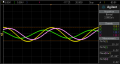With this, Im getting 8.2V @ -37.88 degrees, which seems pretty close to what I want.

The reason why I wasnt doing that was because I couldnt figure out how to make the scope do that. After your last message, I started searching for that function. I found a setting to change it to look from the Math source to Channel 1, instead of Math source to Channel 2.

It seems to be giving me what I would expect. Thanks for your help.

#### WBahn

Joined Mar 31, 2012
26,398
Yep. Now just make sure that you understand why you want Math to Channel 1 and not Channel 1 to Math.

#### Fluke289

Joined Aug 8, 2013
21
Im not quite sure that I do. I wasnt expecting the Math function to give me a wave that was leading Channel 1s wave, so I never thought to think of it that way. I think the correct way to look at it, is that Channel 1 is lagging the Math function by -37.88 degrees.

#### WBahn

Joined Mar 31, 2012
26,398
WBhan you are quite effective with your suggestions but the point is that same scale does not work everywhere..
It is not easy for new ones to use also...
How is that the point? The point here is that it DOES work here, in this case, and this case is what was being discussed. By putting them on the same scale (and 2V/div would have been better, but this was fine), you can immediately see when the two signals are equal and, hence, when the Math signal should be zero. Previously, it was hard to even tell of the Math function was even the difference of the two signals and, if it was, which was being subtracted from which. On the same scale, both of these are immediately obvious.